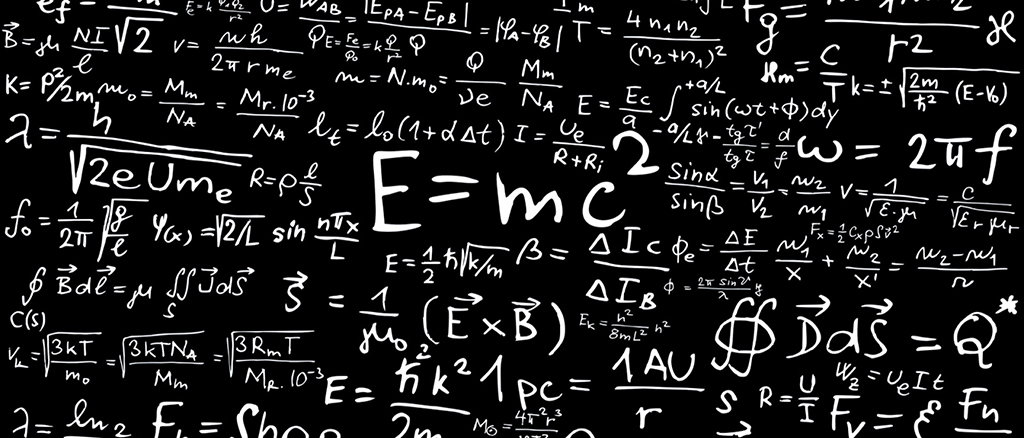# MathJax Example$f\left(a\right)=\frac{1}{2\pi i}{\oint }_{\gamma }\frac{f\left(z\right)}{z-a}dz$

## Configuration

To enable MathJax support, just set plugins.mathjax = true in your theme _config.yml file.

For further MathJax configurations, please edit <theme folder>/layout/plugin/scripts.ejs:

## TeX and LaTeX input

Please be noted that when you write Tex/LaTeX in Markdown files, you need to use escape characters to prevent certain signs from being processed by Markdown interpreter.

#### Result

When $a \ne 0$, there are two solutions to $$ax^2 + bx + c = 0$$ and they are
$$x = {-b \pm \sqrt{b^2-4ac} \over 2a}.$$

## MathML input

Attention: please be noted that newline characters will be transformed to <br> tag by Markdown interpreter and this will interfere with MathML notation. Please write all MathML inline.

#### Result

When$a\ne 0$, there are two solutions to $a{x}^{2}+bx+c=0$ and they are
$x=\frac{-b±\sqrt{{b}^{2}-4ac}}{2a}\text{.}$

## AsciiMath input

Attention: please be noted that when you write AsciiMath in Markdown files, you need to use escape characters to prevent certain signs from being processed by Markdown interpreter.

#### Result

When a != 0, there are two solutions to ax^2 + bx + c = 0 and they are

x = (-b +- sqrt(b^2-4ac))/(2a) .

Share